# Abstracts

Some techniques of multivariate statistical analysis can only be conducted on a complete data matrix, but the process of data collection often misses some elements. Imputation is a technique by which the missing elements are replaced by plausible values, so that a valid analysis can be performed on the completed data set. A multiple imputation method is proposed based on a modification to the singular value decomposition (SVD) method for single imputation, developed by Krzanowski. The method was evaluated on a genotype × environment (G × E) interaction matrix obtained from a randomized blocks experiment on Eucalyptus grandis grown in multienvironments. Values of E. grandis heights in the G × E complete interaction matrix were deleted randomly at three different rates (5%, 10%, 30%) and were then imputed by the proposed methodology. The results were assessed by means of a general measure of performance (Tacc), and showed a small bias when compared to the original data. However, bias values were greater than the variability of imputations relative to their mean, indicating a smaller accuracy of the proposed method in relation to its precision. The proposed methodology uses the maximum amount of available information, does not have any restrictions regarding the pattern or mechanism of the missing values, and is free of assumptions on the data distribution or structure.

missing data; nonparametric; eigenvalue; eigenvector; genotype-environment

dados ausentes; não-paramétrico; autovalor; autovetor; genótipo-ambiente

STATISTICS

Distribution-free multiple imputation in an interaction matrix through singular value decomposition

Imputação múltipla livre de distribuição em matriz de interação por meio de decomposição por valor singular

Genevile Carife BergamoI, II; Carlos Tadeu dos Santos DiasIII, * * Corresponding author < ctsdias@esalq.usp.br> ; Wojtek Janusz KrzanowskiIV

IUNIFENAS - C.P. 23 - 37130-000 - Alfenas, MG - Brasil

IIUSP/ESALQ - Programa de Pós-Graduação em Estatística e Experimentação Agronômica

IIIUSP/ESALQ - Depto. de Ciências Exatas, C.P. 09 - 13418-900 - Piracicaba, SP- Brasil

VISchool of Engineering, Computer Science & Mathematics - University of Exeter -North Park Road - Exeter, EX4 4QE - UK

ABSTRACT

Some techniques of multivariate statistical analysis can only be conducted on a complete data matrix, but the process of data collection often misses some elements. Imputation is a technique by which the missing elements are replaced by plausible values, so that a valid analysis can be performed on the completed data set. A multiple imputation method is proposed based on a modification to the singular value decomposition (SVD) method for single imputation, developed by Krzanowski. The method was evaluated on a genotype × environment (G × E) interaction matrix obtained from a randomized blocks experiment on Eucalyptus grandis grown in multienvironments. Values of E. grandis heights in the G × E complete interaction matrix were deleted randomly at three different rates (5%, 10%, 30%) and were then imputed by the proposed methodology. The results were assessed by means of a general measure of performance (Tacc), and showed a small bias when compared to the original data. However, bias values were greater than the variability of imputations relative to their mean, indicating a smaller accuracy of the proposed method in relation to its precision. The proposed methodology uses the maximum amount of available information, does not have any restrictions regarding the pattern or mechanism of the missing values, and is free of assumptions on the data distribution or structure.

Key words: missing data, nonparametric, eigenvalue, eigenvector, genotype-environment

RESUMO

Palavras-chave: dados ausentes, não-paramétrico, autovalor, autovetor, genótipo-ambiente

INTRODUCTION

Imputation is a technique in which missing elements of a data matrix are replaced by plausible values, so that a valid analysis can be made on the complete data set (observed + imputed). Various imputation methods have been proposed over the years, but the current interest is now focussed mostly on multiple imputation (MI). MI was first proposed by Rubin (1978), and several other references including Little & Rubin (1987, 2002); Rubin (1987); Rubin & Schenker (1986); Schafer (1997, 1999); Tanner & Wong (1987) and Zhang (2003) provide excellent descriptions of the technique. The basic idea of the procedure is to replace each missing value by a set of M imputed values that are drawn from the data distribution, with the variation in these values representing the uncertainty about the true value to be imputed.

The MI procedure involves three distinct steps (i) Imputation: The missing values are estimated M times, generating M completed data sets; (ii) Analysis: The M completed data sets are analyzed, using appropriate statistical procedures for the problem at hand, and (iii) Combination: The M separate sets of results are combined into one single inference.

The imputation is the most critical step, and the model used in this step is not necessarily the same as the one used in the analysis step. This makes the MI procedure more attractive, as the model used to impute data is not always the best one suited for the analysis.

On combining the results of the M analyses, the combined estimative variance consists of the variance within the imputations and, therefore, the uncertainty in the imputed data is incorporated into the final inference.

A method is here proposed for the first step of the multiple imputation, without making any assumptions about the data distribution or structure, by using the singular value decomposition (SVD) of a genotype × environment (G × E) interaction matrix for those models whose analysis needs a complete matrix. The performance of the method is then investigated on randomly deleted entries in the G × E interaction matrix obtained from an experiment on Eucalyptus grandis progenies.

MATERIAL AND METHODS

The data used in this study were obtained from experiments conducted in seven environments, at the south and southeast regions of Brazil, on 20 Eucalyptus grandis progenies obtained from Australia (km 12, South of Ravenshoe-Mt Pandanus-QLD, 43°41'10" W and 22°45'30" S at 33 m above sea level, lot 14,420). A randomized block design with six plants per plot and ten replicates was used, the whole experiment taking up a space of dimension 3.0 m by 2.0 m (Lavoranti, 2003).

The value yij represents the mean tree height (m) over 10 blocks of the plot means for the ith genotype (i = 1,2,...,20) in the jth environment (j = 1,2,...7) of Eucalyptus grandis. The resulting 20 × 7 matrix of yij values constitutes the G × E interaction matrix. Values from this matrix were then deleted randomly to form three different incomplete matrices differing in the proportions of deleted values. The first matrix had 5% deleted, the second 10%, and the third 30%. The same random number seed was used in generating each set of deletions ( ith row; jth column), so that the deleted entries of the second matrix: (6;3), (1;4), (3;4), (6;4), (20;4), (6;5), (7;7) included those deleted in the first: (2;2), (19;2), (10;4), (13;4), (19;4), (5;6), (4;7), and the deleted entries of the third matrix: (3;1), (9;1), (13;1), (15;1), (18;1), (5;2), (11;2), (3;3), (7;3), (17;3), (19;3), (9;4), (12;4), (15;4), (17;4), (8;5), (12;5), (16;5), (2;6), (15;6), (16;6), (17;6), (19;6), (6;7), (8;7), (11;7), (12;7), (13;7), included those deleted in each of the other two. The SAS statistical system, by means of the SAS (2004a) and SAS (2004b), was used in the development of the programs and data analyses described below.

For a distribution free approach, the imputed values were obtained by means of a modification to the simple imputation system developed by Krzanowski (1988). This method starts from the observation made by Good (1969), that any matrix Y(n,p) can be decomposed by the singular value decomposition into the form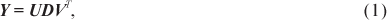in which UTU = VTV = VVT = Ip, UUT = In and D = diag(d1, ..., dp) with d1> d2>,..., > dp > 0. The matrices YTY and YYT have the same eigenvalues, and the elements di are the square roots of these eigenvalues; the ith column vi = (vi1,..., vip)T of the Vp×p matrix is the eigenvector corresponding to the ith largest eigenvalue di2 of YTY; while the jth column uj = (u1j,..., unj)T of the Un×p matrix is the eigenvector corresponding to the jth largest eigenvalue dj2 of YYT. The decomposition (1) has its elementwise representation as follows: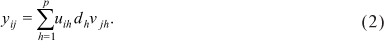Krzanowski (1987) used this representation as a basis for determining the dimensionality of a multivariate data set. If the data structure is essentially H-dimensional then the variation in the remaining (p - H) dimensions can be treated as random noise. The main features of the data can thus be supposed to lie in the space of the first H principal components. The correspondence between the quantities on the right-hand side of (2) and the principal axes of the data configuration suggests, therefore, the H-component model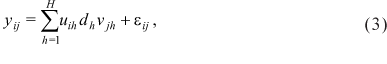in which εij is a residual term.

Supposing that the model (3) holds for a specified value of H, but the single observation yij is missing from the data matrix, then yij can be estimated by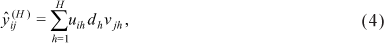in which the uih, dh, vjh, must be estimated from the remaining data. The best estimates of these latter quantities will be those that use the maximal amount of data. Denote by Y (-i) the data matrix obtained on deleting the ith row from Y, and by Y(-j) the data matrix obtained on deleting the jth column from Y, and suppose that the singular value decompositions of these matrices are given by:The maximum-data estimates of uih and vjh in (4) are clearlyand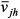, respectively, while dh can be estimated either by,or by some combination of the two. Krzanowski (1988) suggested using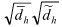as a suitable compromise, so that an estimate of the missing value yij is given by:Following the maximum-data precept, the highest possible value of H should be used. From (6) this is evidently p - 1, so that the value to be imputed for yij will be:An iterative numerical process is needed to find the appropriate quantities in (7). Starting with initial "guesses" for the missing values, each iteration requires singular value decompositions for the reduced matrices Y (-i), Y(-j) for every (i, j) where there is a missing value. The use of (7) provides the updated imputation for that position, and the process continues until convergence (i.e. stability in the successive imputed values). The initial "guesses" for the missing values yij are most easily provided by the mean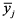of the jth column of the existing values. To avoid any possible variation in the influence among columns, for example caused by different measurement scales, it is recommended to first standardise Y (completed using the initial "guesses"). The Y (-i) and Y(-j) matrices should also be standardised at each step, so that all operations are conducted on standardised quantities. At the end, the completed matrix Y (i.e. observed + imputed values) should be returned to its original scale. Thus if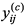represents each value of the completed matrix, the jth column mean ((c)) and standard deviation (sj(c)) are calculated and each value of the completed matrix Y is obtained in its original scale as yij =(c) + sj(c).

The modification to this method that is here proposed is a generalisation to the exponents of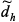andin (7) when generating the imputations (m = 1,..., M) in the first step of the MI. For full generality, if dis represented as a fractional power, we propose changing the exponents in (7) toand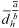subject to the exponents summing to 1 (i.e.). In this way, different weights can be assigned to (5) and (6) in the final estimate of yij in (7), by varying the exponents ofand, whereas the current form forces them to have equal weights.

Each different value ofand consequently ofgenerates a new completed matrix Y, thus providing a mechanism for generating the M different completed data sets at the first step of the multiple imputation process.

The number of imputations is governed by the number of different exponents used. According to Molenberghs & Verbeke (2005); Rubin (1987) and Schafer (1999), between 3 and 5 imputations should be enough to characterise the variability between imputations. Thus, if it is decided on 5 changes in the exponents, between 40% and 60% variation can be produced in the weight given to (5) and (6) by starting with a fixed denominator (b = 20, for instance) and taking values (8, 9, 10, 11 and 12) forand (12, 11, 10, 9 and 8) respectively for. These choices lead to a variation of (40%, 45%, 50%, 55% and 60%) respectively in the proportions of (5) and (6) in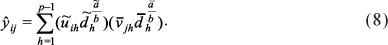The methodology described above and proposed here uses the greatest quantity of values in Y and does not depend on any distribution in the response variable, being applicable to any matrix of numeric data.

Implementation of the method was made by means of a program developed in the IML module of the statistical system SAS, which, after its execution, resulted in a data file with the M = 5 completed data sets, ready to be used in the second step of the MI.

The procedures were applied to the three reduced data matrices, containing 5%, 10% and 30% of missing values. Stability of the imputed values, or their convergence to a single value, was generally achieved within 20 iterations, but, for reasons of security and generality in the program execution, 50 iterations was the default setting. For each percentage of missing values, dispersion graphics were produced for those positions in the data matrix that were randomly deleted at the 5% deletion level. This could be done because the random deletions had the same initial value as seed for all the different percentage deletion levels.

As a measure of performance of the method at missing value position l (falling in row i and column j), the following expression was used:,

adapted from Penny & Jolliffe (1999), in which M is the number of imputations at that missing value position, VOl is the original randomly deleted value at that position, and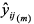is the mth imputation at that position using (8) according to the proposed method. This expression is computed for l = 1,2,...,na, wherena is the total number of missing values. The expression can be separated into two terms,whereis the actual value imputed at position l, so that the first term represents a variance over the M values in each position and the second a bias in the final imputation. Thus the first term is a measure of precision and the second term a measure of accuracy at position l.

An overall measure of performance Tacc may be computed by averaging the accl measures as follows:in which na = g × e × porc, with g representing the total number of genotypes, e representing the total number of environments and porc representing the percentage of missing data.

Tacc may similarly be broken into two components,The first component (VE) represents the pooled variance between imputations within positions, therefore the greater its value is, the smaller is the precision of the multiple imputation method. However, a small value for this component does not necessarily mean that the imputation method is good, for the method may be biased. The second component (VQM) represents the average squared bias between the values of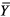and VO, so the smaller the bias is, the bigger is the number of imputations that are similar to the original values and the greater is their accuracy. Therefore, the smaller are the values of VE and VQM, the larger is the multiple imputation method.

RESULTS AND DISCUSSION

Table 1 gives the results for 5%, 10% and 30% of missing values of a random deletion in the matrix. The variability between imputations within each missing value is relatively small, as shown by the small coefficients of variation. Furthermore, this variability increases as the percentage of missing data rises from 5% to 10%, except at the position (19;2) (Figures 1 and 2). When the percentage of missing values increases from 10% to 30%, the variability increases at positions (19;2), (10;4) and (19;4), and it decreases at positions (2;2), (13;4), (5;6) and (4;7) (Figures 2 and 3). The overall precision of multiple imputation is good for these data (Tables 1-2). As for the accuracy, which is the difference between the imputed values and the original values, this shows less consistency than the precision. Values of acc are nearly all larger than their counterparts Var in Table 1, but the pattern is more variable in the other table. So there is no clear relationship between precision and accuracy over the individual missing value positions.

On aggregating over missing value positions, the larger component of the general measure (Tacc) in (10) at all the missing percentages is the average squared bias, see Table 2. However, as the percentage of missing values rises, the variation between imputations defined by expression (11) increases while the average squared bias defined by expression (12) decreases. This results in an improvement of the overall performance, which can be seen through the decrease in the general measure as defined by expression (10).

The proposed method for multiple imputation uses the maximum amount of information available from the original data matrix and is free from assumptions about the distribution or structure of the data. In the G × E interaction matrix for the E. grandis height data, the variability in relation to the mean of imputed values is small, indicating a high precision, and the bias in relation to the original values is also small, indicating good accuracy. However, its values are greater than the variability in relation to the imputed values mean, indicating less accuracy in the proposed model.

ACKNOWLEDGMENTS

To CAPES and CNPq for the award of a scholarship to G.C. Bergamo and C.T.S. Dias, respectively.

Accepted January 07, 2008

• GOOD, I.J. Some applications of the singular value decomposition of a matrix Technometrics, v.11, p.823-831, 1969.
• KRZANOWSKI, W.J. Cross-validation in principal component analysis. Biometrics, v.43, p.575-584, 1987.
• KRZANOWSKI, W.J. Missing value imputation in multivariate data using the singular value decomposition of a matrix. Biometrical Letters, v.25, p.31-39, 1988.
• LITTLE, R.J.; RUBIN, D.B. Statistical analysis with missing data 2nd ed. New York: John Wiley, 1987. 278p.
• LITTLE, R.J.; RUBIN, D.B. Statistical analysis with missing data New York: John Wiley, 2002. 381p.
• MOLENBERGHS, G.; VERBEKE, G. Models for discrete longitudinal data New York: Springer Science, 2005. 683p.
• PENNY, K.I.; JOLLIFFE, I.T. Multivariate outlier detection applied to multiply imputed laboratory data. Statistics in Medicine, v.18, p.1879-1895, 1999.
• RUBIN, D.B. Multiple imputation in sample surveys: a phenomenological Bayesian approach to nonresponce. In: SURVEY RESEARCH METHODS SECTION OF THE AMERICAN STATISTICAL ASSOCIATION, 1978. Proceedings p.20-34. Available at: http://www.amstat.org/sections/srms/Proceedings/ Accessed 20 Nov. 2006.
• RUBIN, D.B. Multiple imputation for nonresponce in surveys New York: John Wiley, 1987. 258p.
• RUBIN, D.B.; SCHENKER, N. Multiple imputation for interval estimation from simple random samples with ignorable nonresponse. Journal of the American Statistical Association, v.81, p.366-374, 1986.
• SAS INSTITUTE. SAS/IML 9.1: user's guide. Carey: SAS Institute, 2004a. 1040p.
• SAS INSTITUTE. SAS/STAT 9.1: user's guide. Carey: SAS Institute, 2004b. 5121p.
• SCHAFER, J.L. Analysis of incomplete multivariate data London: Chapman & Hall, 1997. 430p.
• SCHAFER, J.L. Multiple imputation: a primer. Statistical Methods in Medical Research, v.8, p.3-15, 1999.
• TANNER, M.A.; WONG, W.H. The calculation of posterior distributions by data augmentation (with discussion). Journal of the American Statistical Association, v.82, p.528-550, 1987.
• ZHANG, P. Multiple imputation: theory and method. International Statistical Review, v.71, p.581-592, 2003.
• *
Corresponding author <

# Publication Dates

• Publication in this collection
21 July 2008
• Date of issue
2008